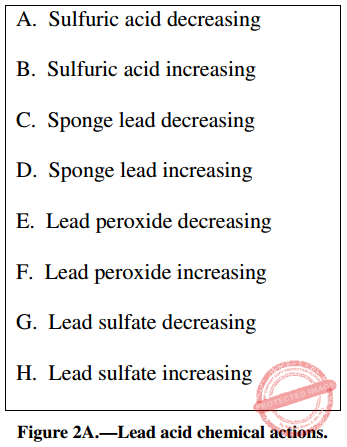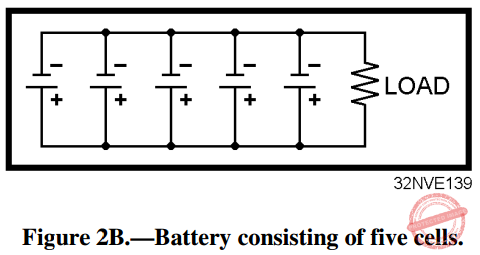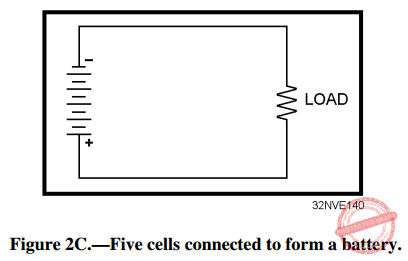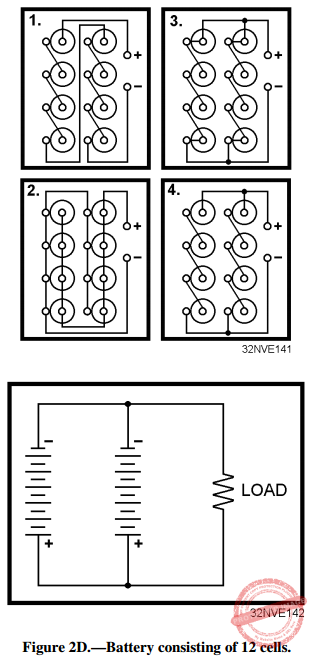# NEETS Module 01 Assignment 2: Batteries

(Last Updated On: December 8, 2017)

This is the Textbook Assignment: Chapter 2, “Batteries” from the Module 1 — Introduction to Matter, Energy, and Direct Current in Navy Electricity and Electronics Training Series (NEETS) . If you are looking for a reviewer in Electronics Engineering this will definitely help. I can assure you that this will be a great help in reviewing the book in preparation for your Board Exam. Make sure to familiarize each and every questions to increase the chance of passing the ECE Board Exam.

### Start Practice Exam Test Questions

Choose the letter of the best answer in each questions.

1. Which of the following is the purpose of an electrical cell?

• A. To change mechanical energy to electrical energy
• B. To change chemical energy to electrical energy
• C. To change electrical energy to mechanical energy
• D. To change electrical energy to chemical energy

2. What are the three basic parts of a cell?

• A. Electrodes, electrolyte, container
• B. Electrodes, acid, water
• C. Anode, cathode, ions

IN ANSWERING QUESTIONS 3 THROUGH 6, SELECT THE PHRASE FROM THE FOLLOWING LIST THAT DESCRIBES THE PART OF A CELL IN THE QUESTION.

I. PARTS OF A CELL

3. Electrolyte

4. Container

5. Anode

6. Cathode

II. DESCRIPTIVE PHRASE

• A. negative electrode
• B. positive electrode
• C. solution acting upon the electrode
• D. mounting for the electrode

7. What term is given to the process that takes place inside a cell?

• A. Electromagnetic action
• B. Piezoelectric action
• C. Electromechanical action
• D. Electrochemical action

8. With respect to recharging a primary or secondary cell, of the following statements, which one is correct?

• A. The secondary cell can be recharged by passing current through it in the proper direction
• B. The primary cell can be recharged by passing current through it in the proper direction
• C. The secondary cell can only be recharged by changing the electrodes
• D. The primary cell can only be recharged by changing the electrolyte

9. What determines the amount of current that a cell can deliver to the external circuit?

• A. The internal resistance of the cell only
• B. The resistance of the external load only
• C. The circuit resistance and the internal resistance of the cell
• D. The circuit capacitance and number of free electrons in the load

10. Which of the following actions will lower the internal resistance of a cell?

• A. Decreasing the size of the electrodes
• B. Increasing the size of the electrodes
• C. Increasing the spacing between the electrodes
• D. Increasing the resistance of the electrolyte

11. What causes negative ions to be attracted to the cathode of a primary cell while the cell is discharging?

• A. A negative charge caused by a loss of electrons
• B. A negative charge caused by an excess of electrons
• C. A positive charge caused by a loss of electrons
• D. A positive charge caused by an excess of electrons

12. What causes hydrogen to be attracted to the anode of a primary cell when the cell is discharging?

• A. A negative charge caused by a loss of electrons
• B. A negative charge caused by an excess of electrons
• C. A positive charge caused by a loss of electrons
• D. A positive charge caused by an excess of electrons

13. What causes the cathode to be "eaten away" in the primary cell while the cell is discharging?

• A. The material of the cathode combines with the negative ions to form a new substance.
• B. The material of the cathode dissolves in the electrolyte.
• C. The material of the cathode leaves the negative terminal of the cell and goes through the load to the anode.
• D. Bacteria in the electrolyte erodes the material in the cathode.

14. The primary cell is completely discharged when which of the following conditions exists?

• A. The cathode is completely eaten away
• B. The active ingredient in the electrolyte is used up
• C. The voltage of the cell is reduced to zero
• D. Each of the above

15. In a zinc-carbon primary cell, what is the function of the carbon electrode?

• A. To generate electrons
• B. To supply a return path for current
• C. To speed electrolysis
• D. To collect hydrogen

16. The lead-acid cell is an example of which of the following types of cells?

• A. The dry cell
• B. The voltaic cell
• C. The primary cell
• D. The secondary cell

17. In a fully charged lead-acid cell, what is the composition of the anode, cathode, and electrolyte respectively?

• A. Zinc, carbon, and water
• B. Carbon, lead, sulfuric acid and water
• D. Nickel, cadmium, potassium hydroxide, and water

18. Which of the following actions will recharge a secondary cell?

• A. Adding more water to the electrolyte
• B. Adding more active ingredient to the electrolyte
• C. Connecting the negative terminal of a voltage source to the cathode of the cell and the positive terminal of the voltage source to the anode of the cell
• D. Connecting the negative terminal of a voltage source to the anode of the cell and the positive terminal of the voltage source to the cathode of the cellIN ANSWERING QUESTIONS 19 AND 20, REFER TO FIGURE 2A. SELECT THE CORRECT CHEMICAL ACTIONS WITHIN A LEAD-ACID CELL FOR THE CONDITION STATED IN EACH QUESTION.

19. The cell is discharging.

• A. A, C, E, H
• B. A, D, E, G
• C. B, C, F, G
• D. B, D, F, H

20. The cell is charging.

• A. A, C, F, H
• B. B, C, F, H
• C. A, D, F, G
• D. B, D, F, G

21. When all the lead sulfate in a lead-acid cell is converted to sulfuric acid, lead peroxide, and sponge lead, what is the condition of the cell?

• A. Fully charged
• B. Discharged
• C. Sulfated
• D. Unusable

22. Polarization has what effects on an electrical cell?

• A. Decreases internal resistance, thereby increasing the output voltage
• B. Decreases internal resistance, thereby decreasing the output voltage
• C. Increases internal resistance, thereby increasing the output voltage
• D. Increases internal resistance, thereby decreasing the output voltage

23. Which of the following methods is used to control polarization in a cell?

• A. Venting the cell
• B. Heating the electrolyte
• C. Adding mercury to the electrode material
• D. Using an electrolyte that absorbs oxygen

24. Which of the following is caused by local action in a cell?

• A. Shelf life is reduced
• B. Hydrogen is generated in large quantities
• C. Impurities rise to the surface of the electrolyte
• D. Mercury coating of the zinc electrode is worn away

25. In a dry cell, what is the consistency of the electrolyte?

• A. Solid
• B. Liquid
• C. Paste
• D. Powder

26. What serves as the cathode in a common type of dry cell?

• A. Carbon electrode
• B. Zinc container
• C. Steel cover
• D. Nickel terminal

27. How should the dry cell be stored to obtain maximum shelf life?

• A. In a dark container
• B. In a heated cabinet
• C. In a ventilated area
• D. In a refrigerated space

28. The blotting paper in a dry cell serves which of the following purposes?

• A. Separates the paste from the zinc
• B. Permits the electrolyte from the paste to filter through to the zinc slowly
• C. Both A and B above
• D. Keeps the electrolyte dry

29. Of the following characteristics, which one describes the mercury cell?

• A. It is physically one of the largest cells
• B. It has a very stable output voltage
• C. It is designed to be rechargeable
• D. It produces a large amount of current but has a short shelf life

30. Which of the following describes the shorting of a cell?

• A. Decreasing the length of a cell
• B. Connecting the anode and cathode together without a load
• C. Using the cell below its full potential
• D. Providing a recharge voltage that is not sufficient to recharge the cell

31. What is/are the advantages(s) of using a manganese-dioxide-alkaline- zinc cell over the zinc-carbon cell?

• A. Better voltage stability
• B. Longer storage life
• C. Operates over a wide temperature range
• D. All the above

32. What is the common name for manganesedioxide-alkaline-zinc cell?

• A. Alkaline cell
• B. Long-life cell
• C. Moz cell
• D. Manganese-dioxide cell

33. Which of the following factors should be considered when selecting a primary cell as a power source?

• A. Power requirement
• B. Type of electrolyte used
• C. Container material
• D. All of the above

34. Of the following types of cells, which one is a primary cell?

• B. Silver zinc
• C. Lithium organic

35. Which of the following is/are the difference(s) in the construction of a NICAD cell as compared to a lead-acid cell?

• A. The electrolyte used
• B. The material of the anode
• C. The material of the cathode
• D. All of the above

36. What is the most common use of a silver-zinc cell?

• A. Flashlight batteries
• B. Automobile batteries
• C. Aircraft storage batteries
• D. Emergency equipment batteries

37. In addition to the nickel-cadmium and silver-zinc cells, which of the following cells uses potassium hydroxide as the active ingredient in the electrolyte?

• C. Lithium-inorganic cell
• D. Magnesium-manganese dioxide cell

38. What is the minimum number of cells necessary to form a battery?

• A. One
• B. Two
• C. Three
• D. FourFigure 2B.—Battery consisting of five cells.

IN ANSWERING QUESTIONS 39 AND 40, REFER TO FIGURE 2B. EACH CELL IS 1.5 VOLTS AND HAS A CAPACITY OF 1/8 AMPERE.

39. What type of connection is used to combine the cells?

• A. Series
• B. Parallel
• C. Series-parallel

40. What is the (a) voltage output and (b) current capacity of the circuit?

• A. (a) 1.5 volts (b) 1/8 ampere
• B. (a) 1.5 volts (b) 5/8 ampere
• C. (a) 7.5 volts (b) 1/8 ampere
• D. (a) 7.5 volts (b) 5/8 ampereFigure 2C.—Five cells connected to form a battery.

IN ANSWERING QUESTIONS 41 AND 42, REFER TO FIGURE 2C. EACH CELL IS 1.5 VOLTS AND HAS A CAPACITY OF 1/8 AMPERE.

41. What type of connection is used to combine the cells?

• A. Series
• B. Parallel
• C. Series-parallel

42. What is the (a) voltage output and (b) current capacity of the circuit?

• A. (a) 1.5 volts (b) 1/8 ampere
• B. (a) 1.5 volts (b) 5/8 ampere
• C. (a) 7.5 volts (b) 1/8 ampere
• D. (a) 7.5 volts (b) 5/8 ampere

43. Which of the following diagrams shows the proper connections for obtaining 6 volts at 1/4 ampere? (Each cell is 1.5 volts and has a capacity of 1/8 amp.)Figure 2D.—Battery consisting of 12 cells.

IN ANSWERING QUESTIONS 44 AND 45, REFER TO FIGURE 2D. EACH CELL EQUALS 1.5 VOLTS AND HAS A CAPACITY OF 1/8 AMPERE.

44. What type of connection is used to combine the cells?

• A. Series
• B. Parallel
• C. Series-parallel

45. What is the (a) voltage output and (b) current capacity of the circuit?

• A. (a) 1.5 volts (b) 1.5 amperes
• B. (a) 4.5 volts (b) 1/2 ampere
• C. (a) 9 volts (b) 1/4 ampere
• D. (a) 18 volts (b) 1/8 ampere

46. What is the first step in performing maintenance on a secondary-cell battery?

• A. Check the level of the electrolyte
• B. Check the technical manual for information on the specific type of battery
• C. Check the terminals for cleanliness and good electrical connection
• D. Check the battery case for cleanliness and evidence of damage

47. When a hydrometer is used to check the specific gravity of the electrolyte in a battery, to what level should the electrolyte be drawn?

• A. Enough to just wet the float
• B. Enough so the float will rise without entering the suction bulb
• C. Enough so the top one-third of the float will rise into the suction bulb
• D. Enough so the float is completely covered by the electrolyte

48. To flush a hydrometer, which of the following liquids should be used?

• A. Sulfuric acid
• B. Salt water
• C. Fresh water
• D. A solution of baking soda and water

49. If the electrolyte level in a battery is low, what should be added to the electrolyte to bring it to the proper level?

• A. Tap water
• B. Sulfuric acid
• C. Potassium hydroxide
• D. Distilled water

50. Which one of the following safety precautions for batteries is NOT correct?

• A. Terminals should be electrically connected together before transporting a battery
• B. Care should be taken to prevent the spilling of electrolyte
• C. Smoking, open flames, and electrical sparks are prohibited around charging batteries
• D. Protective clothing, such as rubber apron, rubber gloves, and face shield, should be worn when working on batteries

51. If electrolyte comes in contact with the skin, what first aid treatment should be given immediately to the affected area?

• A. Cover with petroleum jelly
• B. Wrap with a sterile bandage
• C. Apply an antiseptic lotion
• D. Flush with fresh water

52. A battery with a capacity of 600 ampere-hours should provide 3 amperes for a maximum of how many hours?

• A. 100 hr
• B. 200 hr
• C. 300 hr
• D. 600 hr

53. A battery is rated according to a 20-hour rate of discharge at 300 ampere-hours. Which of the following currents is the maximum current that will allow the battery to deliver its rated capacity?

• A. 15 amperes
• B. 20 amperes
• C. 25 amperes
• D. 30 amperes

54. Which of the following types of routine charges follows the nameplate data in restoring a battery to its charged condition during the ordinary cycle of operation?

• A. Initial
• B. Floating
• C. Normal
• D. Fast

IN ANSWERING QUESTIONS 55 THROUGH 58, MATCH THE DESCRIPTION GIVEN IN THE FOLLOWING LIST WITH THE TYPE OF BATTERY CHARGE IN THE QUESTION.

A. TYPE OF CHARGE

55. Initial charge

56. Equalizing charge

57. Floating charge

58. Fast charge

B. DESCRIPTION

• A. Used in emergency only
• B. Used periodically as part of a maintenance routine
• C. Used to keep a battery at full charge while the battery is idle
• D. Used after electrolyte is added to a dry-shipped battery

59. If violent gassing occurs during the charging of a battery, which of the following actions should be taken?

• A. Increase the room ventilation
• B. Decrease the room temperature
• C. Increase the charging rate
• D. Decrease the charging rate

60. If a battery is being charged at the proper rate, which, if any of the following types of gassing should occur?

• B. Intermittent gassing
• B. Violent gassing
• C. None

Help Me Makes a Difference!

 P inoyBIX educates thousands of reviewers/students a day in preparation for their board examinations. Also provides professionals with materials for their lectures and practice exams. Help me go forward with the same spirit. “Will you make a small \$5 gift today?” Option 1 : \$1 USD Option 2 : \$3 USD Option 3 : \$5 USD Option 4 : \$10 USD Option 5 : \$25 USD Option 6 : \$50 USD Option 7 : \$100 USD Option 8 : Other Amount© 2014 PinoyBIX Engineering. © 2019 All Rights Reserved | How to Donate? |#### GEAS Solution

Dynamics problem Economics problem Physics problem Statics problem Strength problem Thermodynamics problem

#### Questions and Answers in GEAS

Engineering Economics Engineering Laws and Ethics Engineering Management Engineering Materials Engineering Mechanics General Chemistry Physics Strength of Materials Thermodynamics
Consider Simple Act of Caring!: LIKE MY FB PAGE

Our app is now available on Google Play, Pinoybix Elex